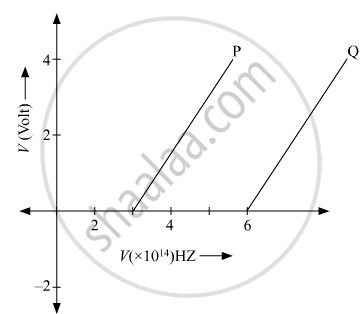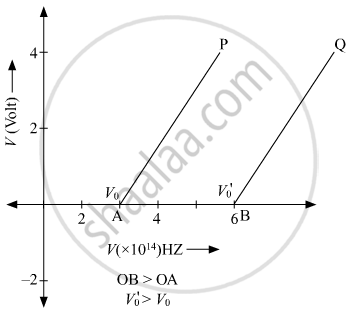Department of Pre-University Education, KarnatakaPUC Karnataka Science Class 12

# In the study of a photoelectric effect the graph between the stopping potential V and frequency v of the incident radiation on two different metals P and Q is shown below - Physics

In the study of a photoelectric effect the graph between the stopping potential V and frequency v of the incident radiation on two different metals P and Q is shown below:(i) Which one of the two metals has higher threshold frequency?

(ii) Determine the work function of the metal which has greater value.

(iii) Find the maximum kinetic energy of electron emitted by light of frequency 8 × 1014 Hz for this metal.

#### SolutionSo the threshold frequency of metal Q is greater than metal P.

(ii)
Work function, w0=hν0

where, v0 is threshold frequency.

0)Q>(ν0)P

⇒WQ>WP

(iii) Maximum kinetic energy is given by

K=E−hν0

WQ>WP

Hence the kinetic energy for metal Q is

E=hν−hν0

= h(v−v0)

=6.63×10−34×(8×1014−6×1014)

=1.33×10−19 J

Concept: Electromagnetic Waves
Is there an error in this question or solution?